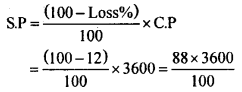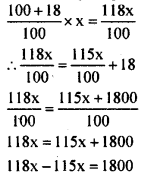# KSEEB Solutions for Class 8 Maths Chapter 9 Commercial Arithmetic Ex 9.2

Students can Download Maths Chapter 9 Commercial Arithmetic Ex 9.2 Questions and Answers, Notes Pdf, KSEEB Solutions for Class 8 Maths helps you to revise the complete Karnataka State Board Syllabus and score more marks in your examinations.

## Karnataka Board Class 8 Maths Chapter 9 Commercial Arithmetic Ex 9.2

Question 1.
Sonu bought a bicycle for Rs. 3750 and spent Rs. 250 on its repairs. He sold it for Rs. 4400. Find his loss or profit percentage.
C.P = 3750 + 250 = Rs. 4000 S P = Rs. 4400
S.P > C.P
∴ S.P – C.P = 4400 – 4000 = Rs. 400
Protit percent =$\frac { profit }{ C.P }$ × 100$\frac { 400}{ 4000 }$ × 100 = 10
Profit percent = 10%

Question 2.
A shop keeper purchases an article for Rs. 3500 and pays the transport charge of Rs. 100. He incurred a loss of 12% in selling this. Find selling price of the article.
C.P = 3500 + 100 = Rs. 3600
Loss percent = 12%S.P = Rs.3168.

Question 3.
By selling a watch for Rs. 720, Ravi loses 10%. At what price should he sell it, in order to gain 15% .
S.P = Rs. 720,
loss percent = 10% C.P = ?
\begin{aligned} \mathrm{CP} &=\frac{100}{(100-\text { Loss percent })} \times \mathrm{SP} \\ &=\frac{100}{(100-10)} \times 720=\frac{100}{90} \times 720 \end{aligned}
C.P = RS. 800
gain percent = 15 %
S.P = ?
\begin{aligned} \mathrm{S.P} &=\frac{(100+\mathrm{gain} \%)}{100} \times \mathrm{C.P} \\ &=\frac{100+15}{100} \times 800=\frac{115}{100} \times 800 \end{aligned}
S.P = Rs. 920
He should sell it for Rs. 920 to gain a 15% profit.

Question 4.
Hari bought two fans for Rs. 2400 each. He sold one at a loss of 10% and the other at a profit of 15%. Find the selling price of each fan and also the total profit or loss.
C.P = Rs. 2400, Loss% = 10%
S.P = ?S.P = Rs. 2160
∴ When the loss percent is 10% S.P. is Rs. 2160
C.P = Rs. 2400
profit percent = 15%
S.P = ?
\begin{aligned} \mathrm{S.P} &=\frac{(100+\text { Profit } \%)}{100} \times \mathrm{C.P} \\ &=\frac{100+15}{100} \times 2400=\frac{115}{100} \times 2400 \end{aligned}
S.P. = Rs. 2760.
When the profit percent is 15%, the S.P. is Rs. 2760.
Total C.P. of two fans is 2400 + 2400 = Rs. 4800.
Total S.P. of two fans is 2160 + 2760 = Rs. 4920.
Total SP > Total C.P
∴ Total profit = Total S.P – Total C.P = 4920-4800 Profit = Rs. 120.

Question 5.
A storekeeper sells a book at a 15% gain. Had he sold it for Rs. 18 more. He would have gained 18%. Find the cost price of the book.
Let the C.P be Rs. x
gain % = 15%Had he sold it for Rs. 18 more the new S.P.
would be Rs. $$\frac{115 x}{100}$$ . This is equal to S.P
When the gain percent is 18%
S.P when gain percent is 18% is118 x – 115 x = 1800
3 x = 1800
x = $$\frac { 1800 }{ 3 }$$
x = 600
∴ Cost price = Rs.600.

Question 6.
The cost price of 12 pens is equal to the selling price of 10 pens. Find the profit percentage.
Gain percent = $$\frac { Gain }{ C.P }$$ × 100
= $$\frac { 2 }{ 10 }$$ × 100 = 20 %